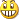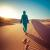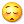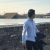# 简单而直接的Python web 框架：web.py

web.py 是一个Python 的web 框架，它简单而且功能强大。web.py 是公开的，无论用于什么用途都是没有限制的。

`import weburls = (    '/(.*)', 'hello')class hello:    def GET(self, name):        i = web.input(times=1)        if not name: name = 'world'        for c in xrange(int(i.times)): print 'Hello,', name+'!'if __name__ == "__main__": web.run(urls, globals())`

1. 安装

2. URL 处理

`import web`

`urls = (  '/', 'index'    )`

GET 是最普遍的方法，用来请求一个页面。当我们在浏览器里输入“harvard.edu” 的时候，实际上它是向Web 服务器请求GET ”/“。另一个常见的方法是POST，常用于提交特定类型的表单，例如利用信用卡付费和处理一个订单。注意，GET URLs 能够被搜索引擎索引得到（想像一下Google 尝试购买你网站上的所用物品）。

`class index:    def GET(self):        print "Hello, world!"`

`if __name__ == "__main__": web.run(urls, globals())`

`import weburls = (  '/', 'index'    )class index:    def GET(self):        print "Hello, world!"if __name__ == "__main__": web.run(urls, globals())`

``\$ python code.pyLaunching server: http://0.0.0.0:8080/``

3. 调试

web.py 本身也提供调试的工具。在最后的“if name …” 代码前面添加：

``web.webapi.internalerror = web.debugerror``

``if __name__ == "__main__": web.run(urls, globals(), web.reloader)``

4. 模板

``<em>Hello</em>, world!``

``\$def with (name)\$if name:    I just wanted to say <em>hello</em> to \$name.\$else:    <em>Hello</em>, world!``

``render = web.template.render('templates/')``

`def GET(self):    name = 'Bob'    print render.index(name)`

`urls=(    `'/(.*)', 'index')``

`def GET(self,name):    print render.index(name)`

5. 数据库

``web.config.db_parameters = dict(    dbn='postgres',``    ``user='username',``    ``pw='password',``    ``db='dbname')``

``CREATE TABLE todo (  id serial primary key,  title text,  created timestamp default now(),  done boolean default 'f');``

``INSERT INTO todo (title) VALUES ('Learn web.py');``

``def GET(self):    todos = web.select('todo')    print render.index(todos)``

``urls = (`    `'/', 'index')``

``\$def with (todos)<ul>\$for todo in todos:    <li id="t\$todo.id">\$todo.title</li></ul>``

``<form method="post" action="add">   <p>       <input type="text" name="title" />       <input type="submit" value="Add" />   </p></form>``

``urls = (    ``'/', 'index',    '/add', 'add')``

``class add:    def POST(self):        i = web.input()        n = web.insert('todo', title=i.title)        web.seeother('/')``

web.input 使你能够方便地访问用户通过表单提交上来的变量。web.insert 用于向数据库的 “todo” 表插入数据，并且返回新插入行的ID。web.seeother 用于重转向到”/“。

6. 总结

web.py 的确相当的小巧，应当归属于轻量级的web 框架。但这并不影响web.py 的强大，而且使用起来很简单、很直接。在实际应用上，web.py 更多的是学术上的价值，因为你可以看到更多web 应用的底层，这在当今“抽象得很好”的web 框架上是学不到的 ：） 如果想了解更多web.py，可以访问web.py 的官方文档

3勘误勘误～

if __name__ == "__main__":
app = web.application(urls, globals())
app.run()

0
w

0`http://0.0.0.0:8080/`

http://localhost:8080/0web.py很强大，而且也很简单。我现在就在使用它。
0
m

web 3.x下，第一段代码运行出错，修改为：

import web

urls = ( '/(.*)', 'hello',)

class hello(object):
def GET(self, name):
i = web.input(times=1)
if not name:name = 'world'
for c in xrange(int(i.times)):
return 'Hello, %s !'% name

if __name__ == '__main__':
app = web.application(urls, globals())
app.run()